# Texas Go Math Grade 1 Lesson 1.2 Answer Key Make Ten and Ones

Refer to our Texas Go Math Grade 1 Answer Key Pdf to score good marks in the exams. Test yourself by practicing the problems from Texas Go Math Grade 1 Lesson 1.2 Answer Key Make Ten and Ones.

## Texas Go Math Grade 1 Lesson 1.2 Answer Key Make Ten and Ones

Essential Question
How can you show a number as ten and ones?
Explanation
By using the ten frame we can say tens and ones
Explanation:
By making to 10 frame
14 in 3 different ways
1 tens 4 ones
10 + 4 = 14
10 ones 4 ones

Explore
Use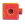to model the problem.
Drawto show your work.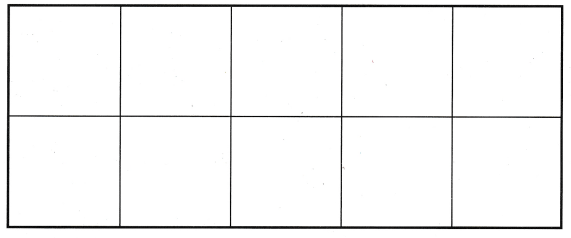Draw to show the group of ten another way.For The Teacher
Read the problem. Connie has 10 cubes. How can she arrange them to show 1 ten?
Explanation:
1 ten frame has 10 ones cubesMath Talk
Mathematical Processes
How are the pictures the same? How are the pictures different? Explain.
Explanation:
The pictures are same because 10 ones = 1 tens

Model and Draw

You can group 10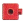to make 1 ten.

Draw a quick picture to show ten.

You can group 10to make 1 ten.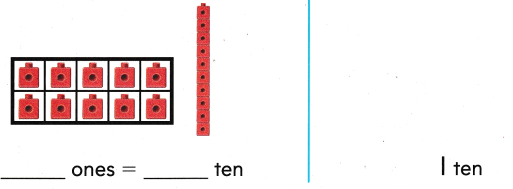Explanation: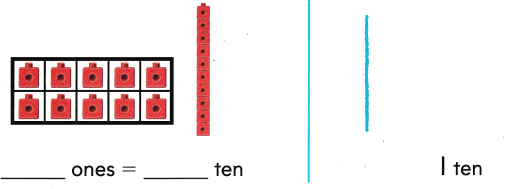10 ones = 1 tens
By grouping 10 ones cubes to make 1 ten

Share and Show

Use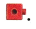Make groups of ten and ones. Draw your work. Write how many.

Question 1.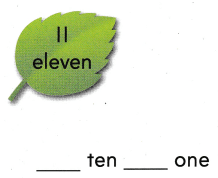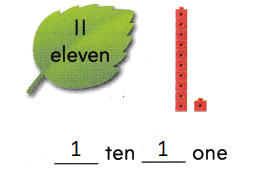Explanation:
the sum of 1 tens 1 ones = 11
10 + 1 = 11

Question 2.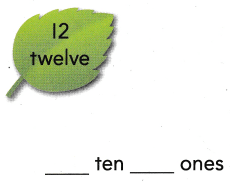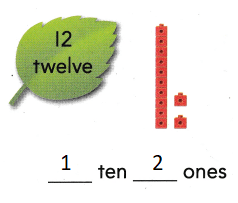Explanation:
the sum of 1 tens 2 ones
10 + 2 = 12

Question 3.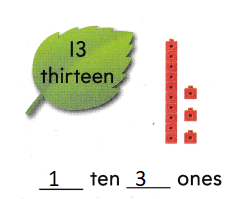Explanation:
the sum of 1 tens 3 ones
10 + 3 = 13

Question 4.Explanation:
the sum of 1 tens 4 ones
10 + 4 = 14

Problem Solving
UseMake groups of ten and ones. Draw your work. Write how many.

Question 5.Explanation:
the sum of 1 tens 5 ones
10 + 5 = 15

Question 6.Explanation:
the sum of 1 tens 6 ones
10 + 6 = 16

Solve.

Question 7.
Emily wants to write ten and ones to show 20. What does Emily write?
___ ten ___ ones1 tens 10 ones
10 + 10 = 20
Explanation: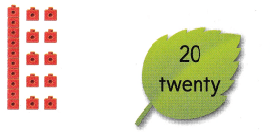Question 8.
Multi-Step Carter has 5 ones. Mia has 5 ones. Logan has 3 ones. They put all their ones together. Draw a quick picture to show how many tens and ones they made. Write how many.
_____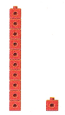Explanation:
Carter has 5 ones. Mia has 5 ones. Logan has 3 ones.
5 + 3 + 3 = 11
1 tens and 1 ones

Question 9.
Kiley has 1 ten. Chloe has 5 ones. They put the ten and ones together. Which shows how many they have altogether?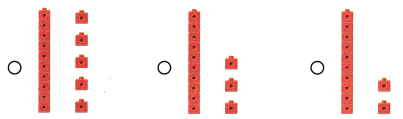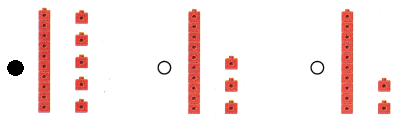Explanation:
10 + 5 = 15
15 have altogether

Question 10.
Use Tools Matt drew a picture to show a number. What is the number?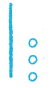(A) 13
(B) 14
(C) 15
Explanation:
1 line = 10 and 1 dot = 1 ones
10 + 3 = 13

Question 11.
Multi-Step 10 friends are having dinner. 5 more friends join them. Then Pete and Julia join their friends. Which shows how many friends are having dinner?
(A) 1 ten 2 ones
(B) 1 ten 5 ones
(C) 1 ten 7 ones
10 friends are having dinner.
5 more friends join them
10 + 5 =15
15 + 2 = 17
1 ten 7 ones  shows that how many friends are having dinner

Question 12.
Texas Test Prep How many tens and ones make this number?
(A) 1 ten 2 ones
(B) 2 tens 1 one
(C) 1 tens 12 ones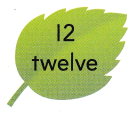Explanation:
1 ten 2 ones make number 12

Take Home Activity

• Give your child numbers from 11 to 19. Have your child work with pennies to show a group of ten and a group of ones for each number.
10 + 1 = 11
1 tens and 2 ones
10 + 2 = 12
1 tens and 3 ones
10 + 3 = 13
1 tens and 4 ones
10 + 4 = 14
1 tens and 5 ones
10 + 5 = 15
1 tens and 6 ones
10 + 6 = 16
1 tens and 7 ones
10 + 7 = 17
1 tens and 8 ones
10 + 8 = 18
1 tens and 8 ones
10 + 9 = 19
1 tens and 9 ones

### Texas Go Math Grade 1 Lesson 1.2 Homework and Practice Answer Key

Use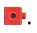Make groups of ten and ones. Draw your work. Write how many.

Question 1.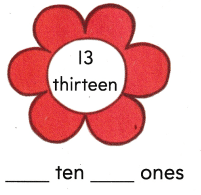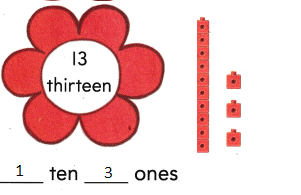Explanation:
the sum of 1 tens  3 ones
10 + 3 = 13

Question 2.Explanation:
the sum of 1 tens 8 ones
10 + 8 = 18

Solve.

Question 3.
Kim wants to write ten and ones to show 19? What does Kim write?
____ ten ___ ones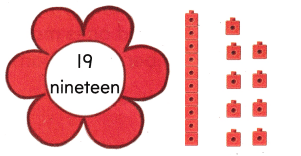Explanation:
the sum of 1 tens 9 ones
is 19
10 + 9 = 19

Texas Test Prep

Lesson Check

Question 4.
A flower had 14 petals. Which shows how many petals the flower had?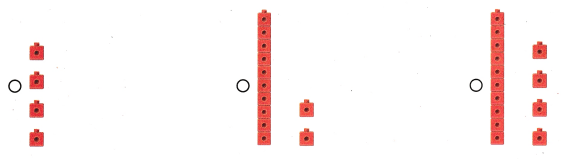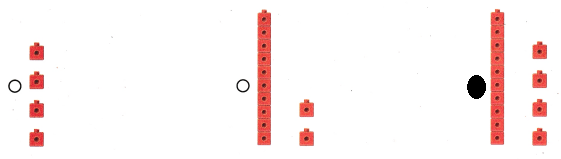Explanation:
1 tens and 4 ones
10 + 4 = 14

Question 5.
10 leaves fell off a tree. How many tens and ones are in 10?
(A) 1 ten 0 ones
(B) 0 tens 1 ones
(C) 1 ten 1 ones
Explanation:
1 ten 0 ones  are in 10
10 + 0 = 10

Question 6.
Chen drew a picture to show a number. What is the number?(A) 1 ten 2 ones
(B) 1 ten 8 ones
(C) 1 ten 5 ones